# Selina Solutions Concise Mathematics Class 6 Chapter 31 Recognition of Solids

Selina Solutions Concise Mathematics Class 6 Chapter 31 Recognition of Solids provides basic knowledge among students, as per the current ICSE exam guidelines. The solutions prepared mainly boost confidence among students in solving complex problems. Frequent practice of these solutions improves their time management skills to face the examination fearlessly. To get their doubts cleared instantly, students can use Selina Solutions Concise Mathematics Class 6 Chapter 31 Recognition of Solids PDF, from the links which are provided below

Chapter 31 deals with the study of important concepts such as identification of 3D shapes and nets, which can be used to form cubes. Students can use these solutions to understand the skills of solving problems with ease.

## Selina Solutions Concise Mathematics Class 6 Chapter 31 Recognition of Solids Download PDF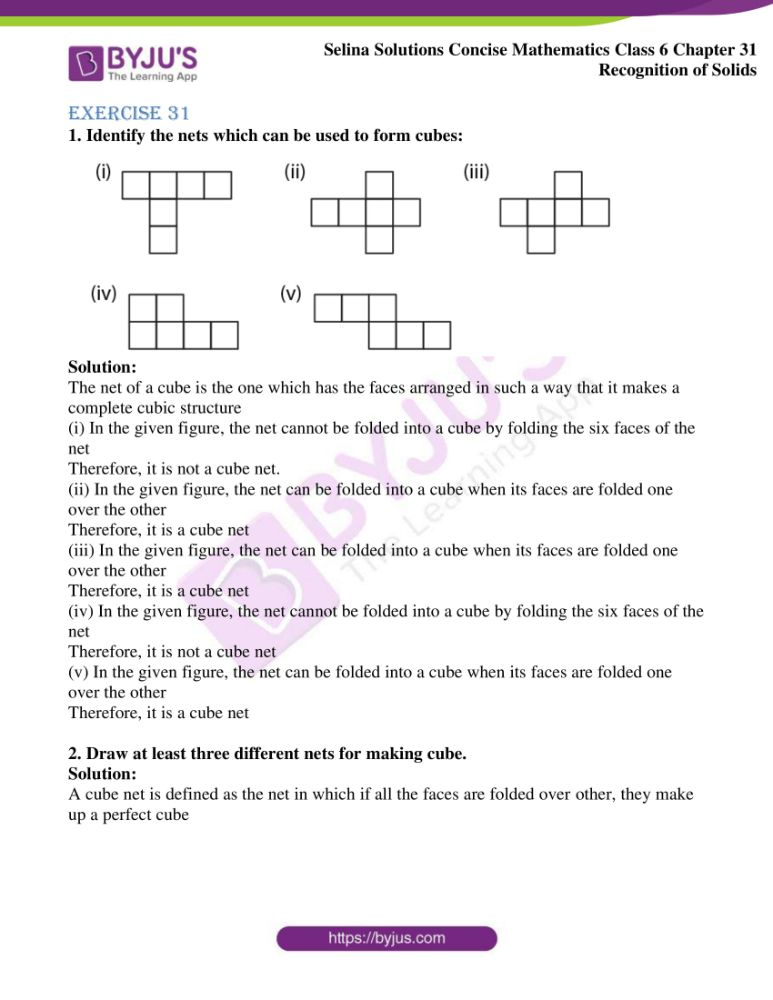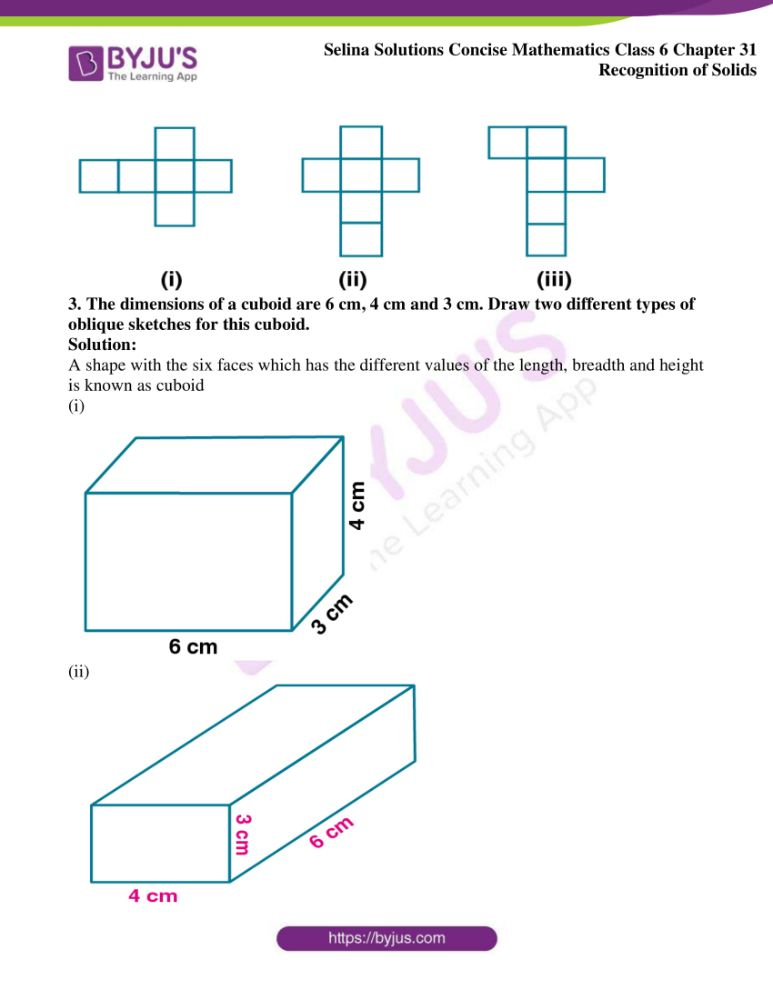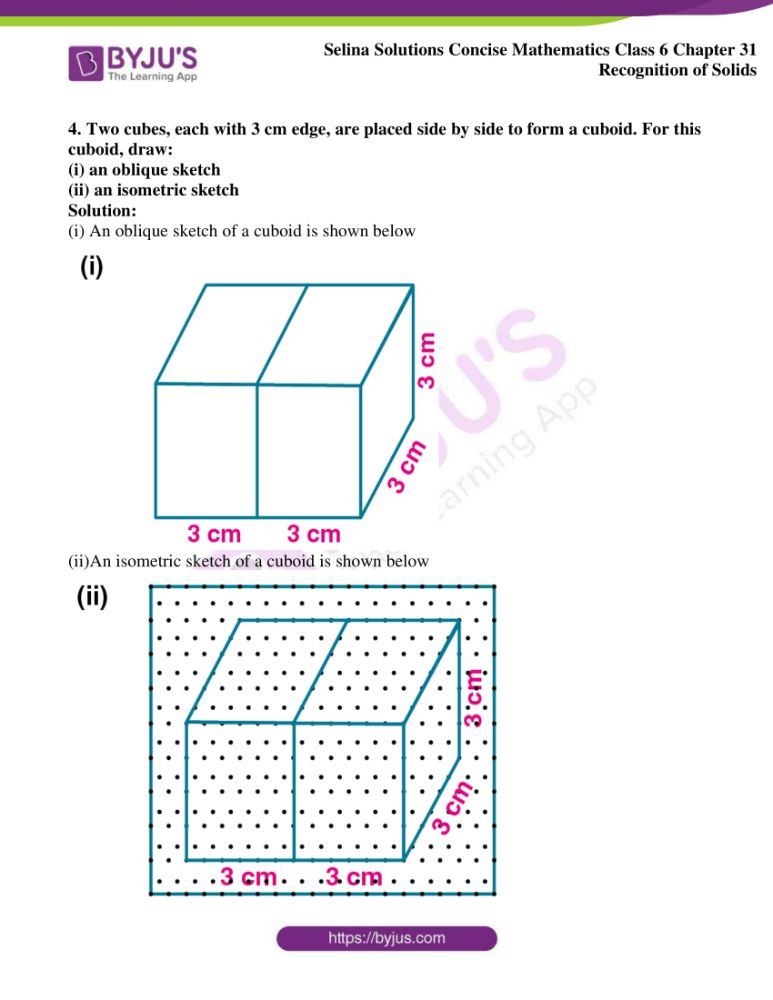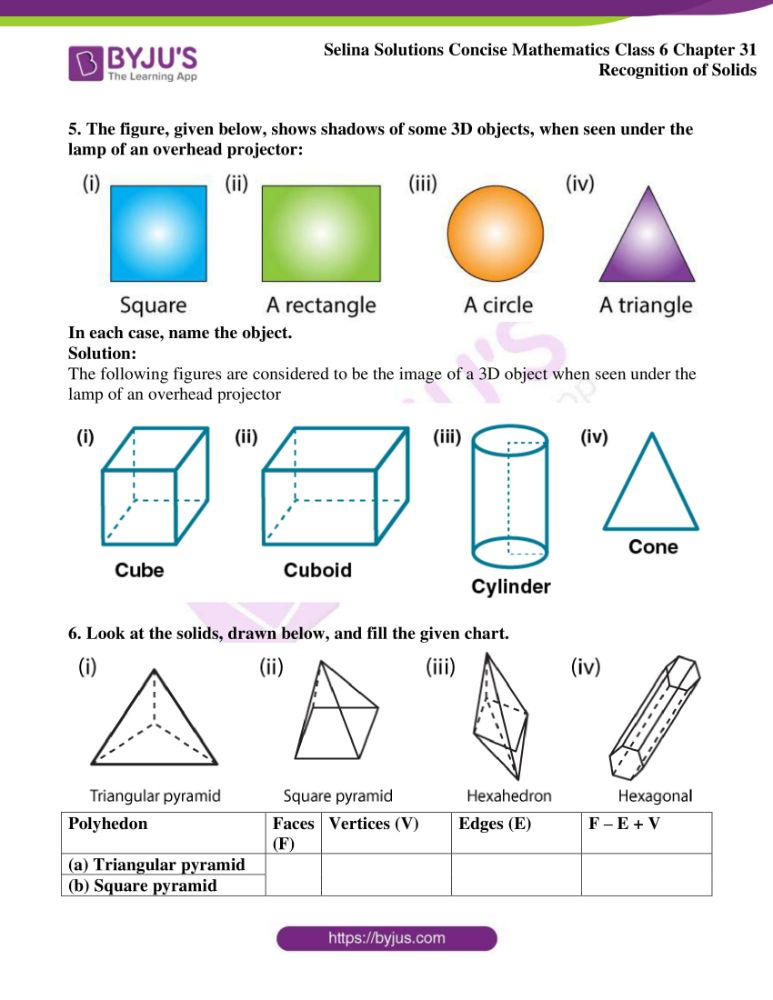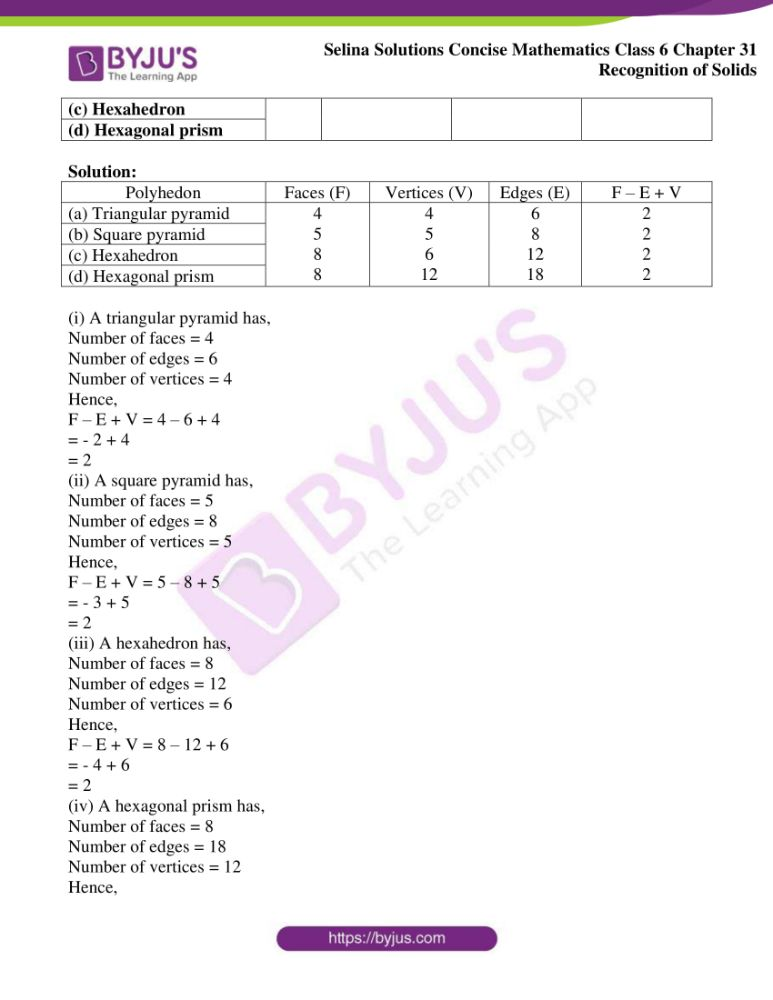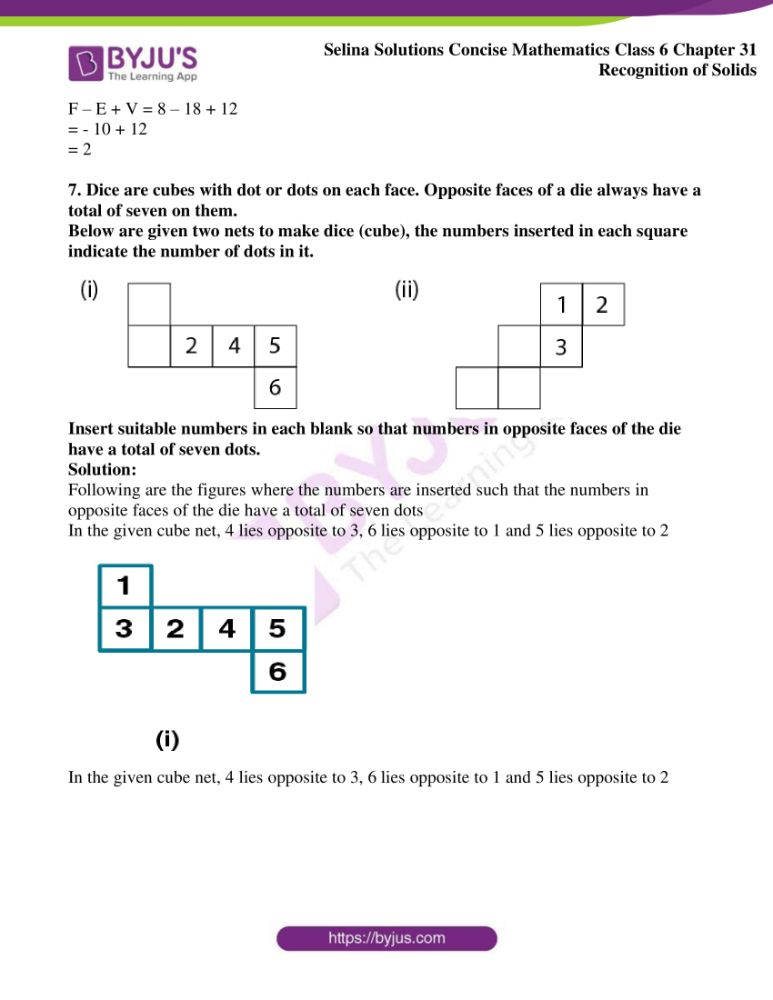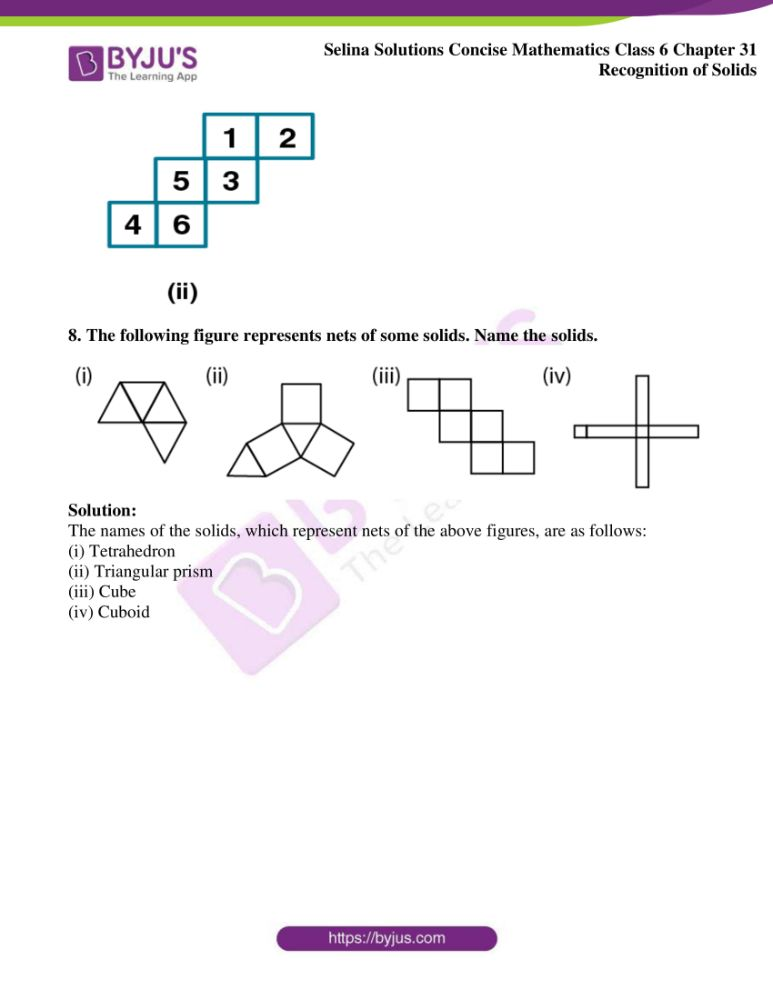## Access Selina Solutions Concise Mathematics Class 6 Chapter 31: Recognition of Solids

Exercise 31

1. Identify the nets which can be used to form cubes: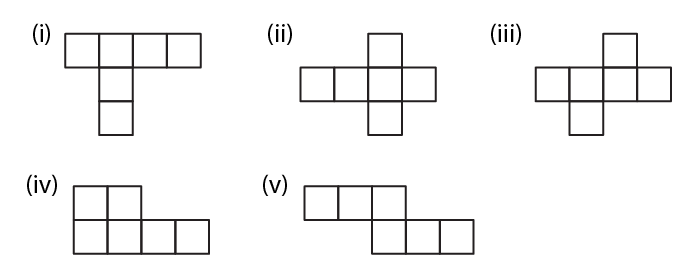Solution:

The net of a cube is the one which has the faces arranged in such a way that it makes a complete cubic structure

(i) In the given figure, the net cannot be folded into a cube by folding the six faces of the net

Therefore, it is not a cube net.

(ii) In the given figure, the net can be folded into a cube when its faces are folded one over the other

Therefore, it is a cube net

(iii) In the given figure, the net can be folded into a cube when its faces are folded one over the other

Therefore, it is a cube net

(iv) In the given figure, the net cannot be folded into a cube by folding the six faces of the net

Therefore, it is not a cube net

(v) In the given figure, the net can be folded into a cube when its faces are folded one over the other

Therefore, it is a cube net

2. Draw at least three different nets for making cube.

Solution:

A cube net is defined as the net in which if all the faces are folded over other, they make up a perfect cube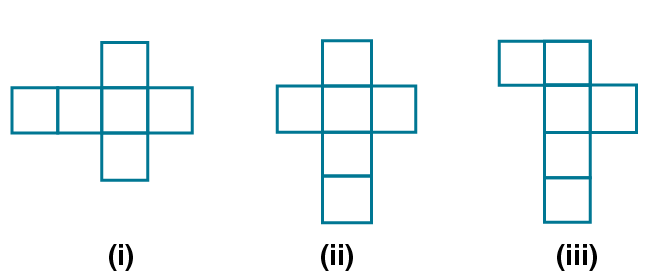3. The dimensions of a cuboid are 6 cm, 4 cm and 3 cm. Draw two different types of oblique sketches for this cuboid.

Solution:

A shape with the six faces which has the different values of the length, breadth and height is known as cuboid

(i)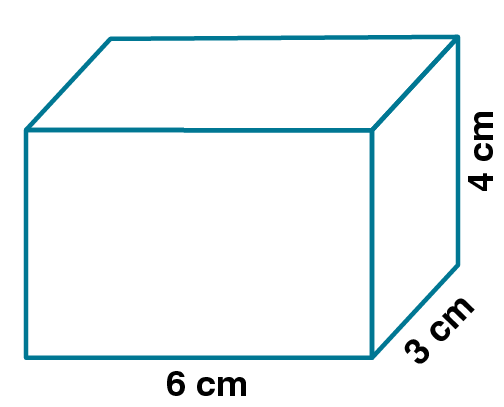(ii)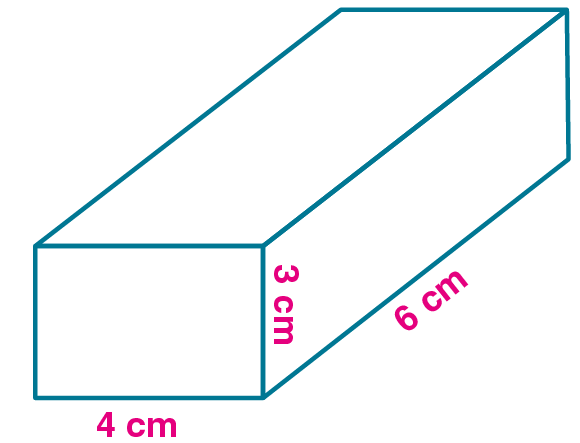4. Two cubes, each with 3 cm edge, are placed side by side to form a cuboid. For this cuboid, draw:

(i) an oblique sketch

(ii) an isometric sketch

Solution:

(i) An oblique sketch of a cuboid is shown below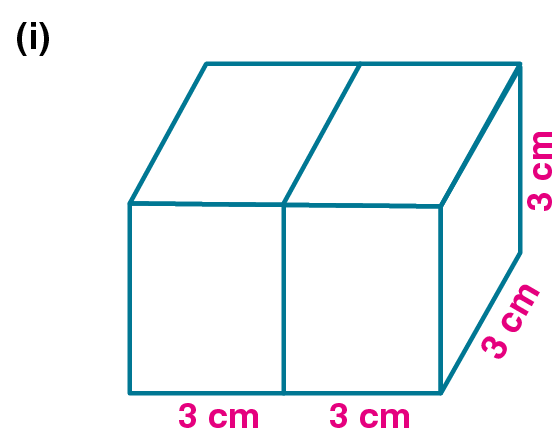(ii)An isometric sketch of a cuboid is shown below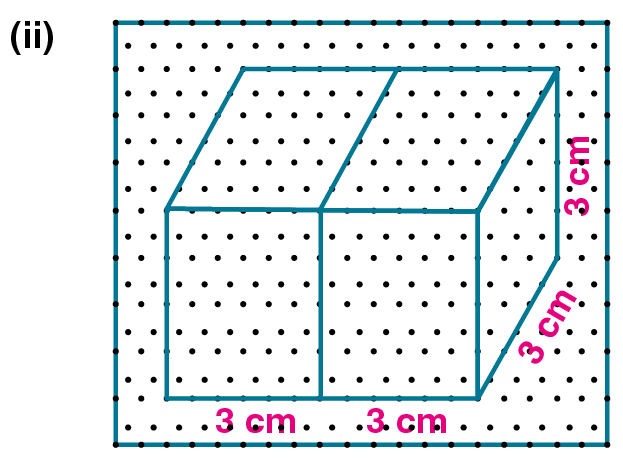5. The figure, given below, shows shadows of some 3D objects, when seen under the lamp of an overhead projector: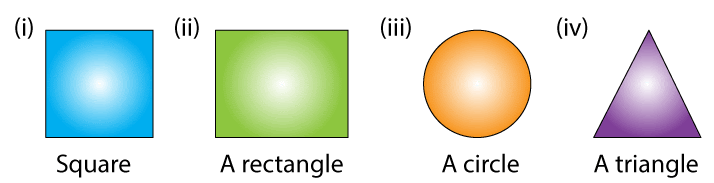In each case, name the object.

Solution:

The following figures are considered to be the image of a 3D object when seen under the lamp of an overhead projector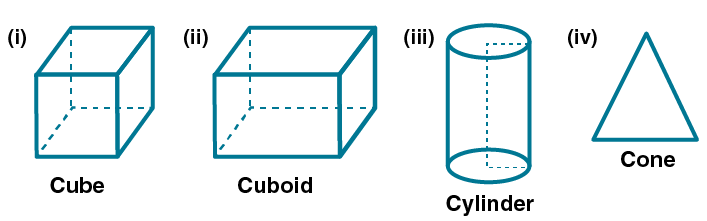6. Look at the solids, drawn below, and fill the given chart.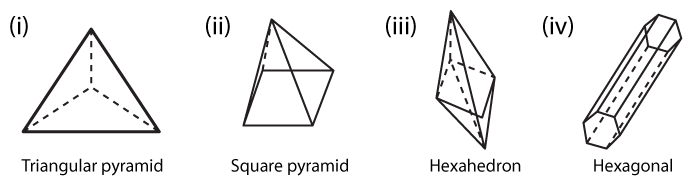Polyhedon Faces (F) Vertices (V) Edges (E) F – E + V (a) Triangular pyramid (b) Square pyramid (c) Hexahedron (d) Hexagonal prism

Solution:

 Polyhedon Faces (F) Vertices (V) Edges (E) F – E + V (a) Triangular pyramid 4 5 8 8 4 5 6 12 6 8 12 18 2 2 2 2 (b) Square pyramid (c) Hexahedron (d) Hexagonal prism

(i) A triangular pyramid has,

Number of faces = 4

Number of edges = 6

Number of vertices = 4

Hence,

F – E + V = 4 – 6 + 4

= – 2 + 4

= 2

(ii) A square pyramid has,

Number of faces = 5

Number of edges = 8

Number of vertices = 5

Hence,

F – E + V = 5 – 8 + 5

= – 3 + 5

= 2

(iii) A hexahedron has,

Number of faces = 8

Number of edges = 12

Number of vertices = 6

Hence,

F – E + V = 8 – 12 + 6

= – 4 + 6

= 2

(iv) A hexagonal prism has,

Number of faces = 8

Number of edges = 18

Number of vertices = 12

Hence,

F – E + V = 8 – 18 + 12

= – 10 + 12

= 2

7. Dice are cubes with dot or dots on each face. Opposite faces of a die always have a total of seven on them.

Below are given two nets to make dice (cube), the numbers inserted in each square indicate the number of dots in it.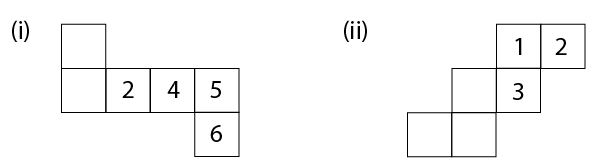Insert suitable numbers in each blank so that numbers in opposite faces of the die have a total of seven dots.

Solution:

Following are the figures where the numbers are inserted such that the numbers in opposite faces of the die have a total of seven dots

In the given cube net, 4 lies opposite to 3, 6 lies opposite to 1 and 5 lies opposite to 2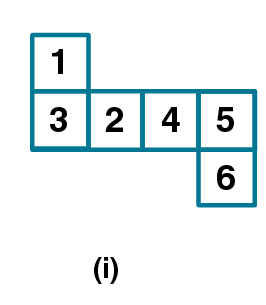In the given cube net, 4 lies opposite to 3, 6 lies opposite to 1 and 5 lies opposite to 2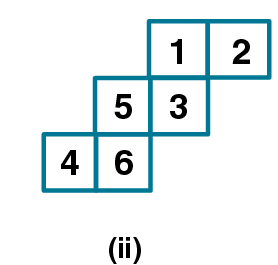8. The following figure represents nets of some solids. Name the solids.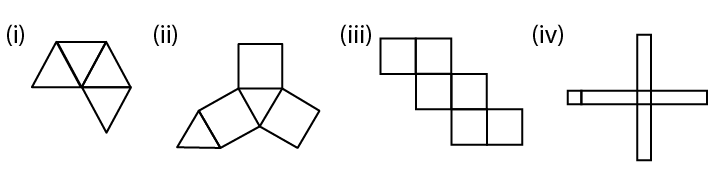Solution:

The names of the solids, which represent nets of the above figures, are as follows:

(i) Tetrahedron

(ii) Triangular prism

(iii) Cube

(iv) Cuboid